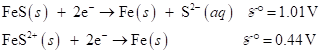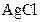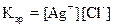Chapter 18, Problem 89E

Chapter
Section
Textbook Problem

# Calculate Ksp for iron(II) sulfied given the following data:Interpretation Introduction

Interpretation:

Ksp of given FeS should be calculated.

Concept introduction:

Electromotive force:

The potential difference between two electros in the galvanic cell is known as electromotive force (EMF) and it is given by

Ecell=Ecathode-Eanode

The loss of electron is oxidation and this is occurs in anode and gain of electron is reduction and this is occurs in cathode.

If the EMF is positive the cell reaction will take place spontaneously, if the EMF is negative the cell reaction will not take place spontaneously.

Solubility product:means the solubility product constant which is nothing but a equilibrium constant for salts that do not dissolve fully in a solvent.  It is the product of the dissolved ion concentrations of a salt with their stoichiometric coefficients raised as its power.

The equilibrium expression for the dissociation ofsalt is given as,When thevalue will be small, the solubility will be less

Explanation

Given,

FeS+2e-Fe(s) +S2-(aq)=-1.01VFe2+(aq)+2e-Fe(s)    E°=-0.44V

Consider the highest positive value is cathode reaction.

The EMF of the cell is calculated below,

Ecell=Ecathode-Eanode=-0.44V-1.01V=-1

### Still sussing out bartleby?

Check out a sample textbook solution.

See a sample solution

#### The Solution to Your Study Problems

Bartleby provides explanations to thousands of textbook problems written by our experts, many with advanced degrees!

Get Started